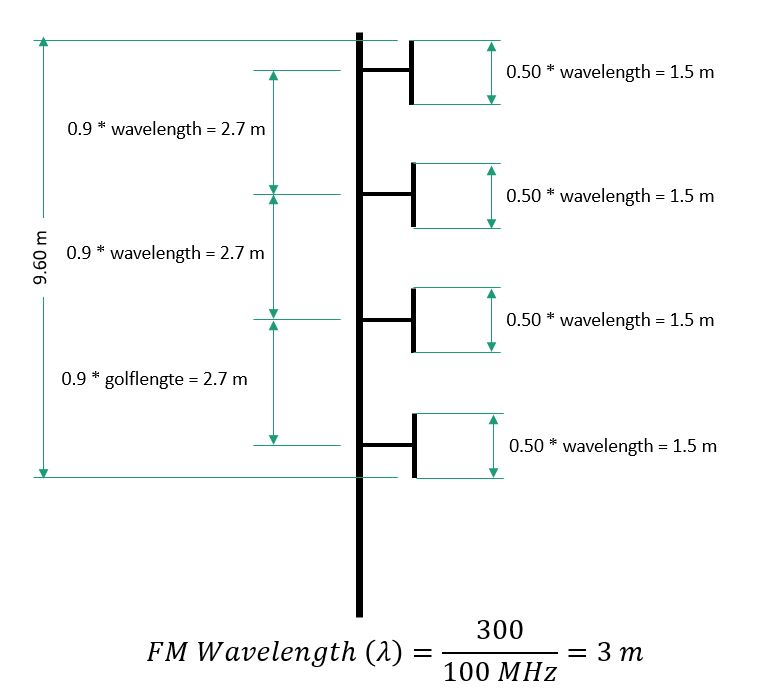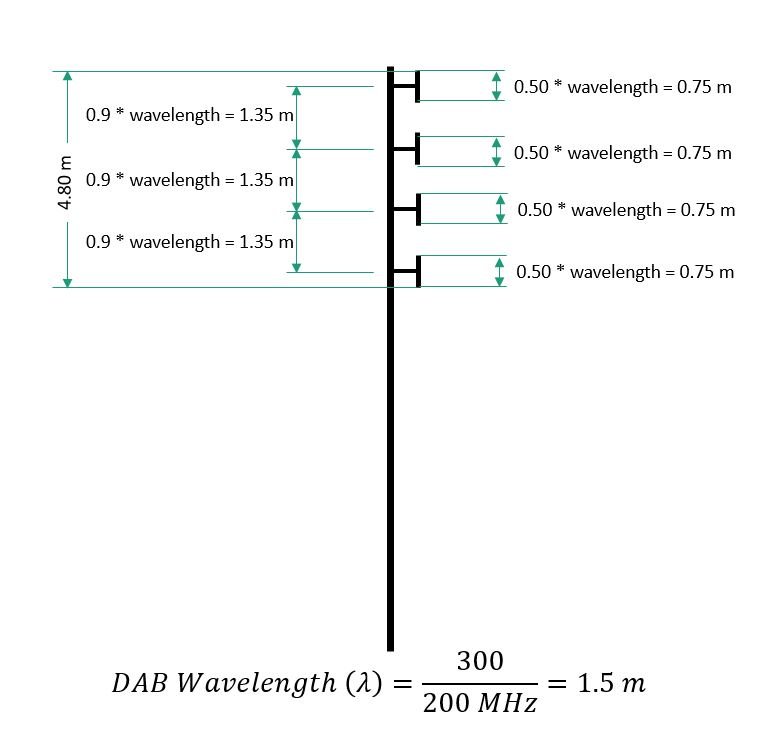# The DAB+ antenna

The antenna used for DAB(+) is shorter than the antenna for FM. The reason for this is the higher frequency band in which DAB(+) (MODE I) is operating. The speed of displacement of an electromagnetic wave is a constant speed (speed of light), the wavelength of the wave is inversely proportional to the frequency. Which results in the higher the frequency, the smaller the wavelength. The length of the antenna is always related to the wavelength. A higher frequency logically means a shorter antenna.

An half-wave dipole is, as the description says, half a wavelength long (provided small differences due to the speed of the radio wave which is slightly less in air than in vacuum and also depends on the type of metal from which the antenna is made). Roughly one can calculate the wavelength by dividing the speed of light [m/s] by the frequency (Hz). Easiest way is to divide 300 by the frequency in MHz to become the wavelength in meters.

Divide 300 by the frequency in MHz to become the wavelength in m

In the middle of the FM-band (VHF Band-II) the frequency is 98 MHz, which corresponds to a wavelength of 300/98 3.00 meters. A half wavelength is then 1.5m. In the middle of the DAB band (VHF Band-III) the frequency is about 200 MHz, which in turn corresponds to a wavelength of 300/200 = 1.5 meters. A half wave is then 0.75 m.

A DAB dipole will therefore be half the size of an FM dipole.

See the following drawings comparing FM- and DAB/DAB+ antennas.The distance between two dipoles, approximately 0.9 times the wavelength, will also decrease: from 2.7 meters (FM) to 1.35 meters for DAB(+). The total length of a properly placed FM antenna with 4 dipoles will have a total height of 9.60 meters (3 times 2.7 meters, plus the length of one dipole antenna being 1.5 m).

If one calculates in the same way 4 dipoles one above the other for a DAB(+) antenna, one arrives at 4.8 m (3 times 1.35 m, plus the length of one dipole antenna being 0.75 m). A 4 dipole antenna for DAB(+) is therefore half the size of an antenna for FM. Anyway, this makes mounting much easier than for a FM antenna.

Back